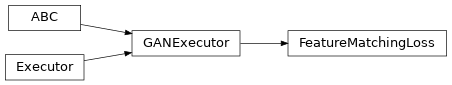# FeatureMatchingLoss¶

Inheritance Diagramclass ashpy.losses.gan.FeatureMatchingLoss[source]

Conditional GAN Feature matching loss.

The loss is computed for each example and it’s the L1 (MAE) of the feature difference. Implementation of pix2pix HD: https://github.com/NVIDIA/pix2pixHD

$\text{FM} = \sum_{i=0}^N \frac{1}{M_i} ||D_i(x, c) - D_i(G(c), c) ||_1$

Where:

• D_i is the i-th layer of the discriminator

• N is the total number of layer of the discriminator

• M_i is the number of components for the i-th layer

• x is the target image

• c is the condition

• G(c) is the generated image from the condition c

• || ||_1 stands for norm 1.

This is for a single example: basically for each layer of the discriminator we compute the absolute error between the layer evaluated in real examples and in fake examples. Then we average along the batch. In the case where D_i is a multidimensional tensor we simply calculate the mean over the axis 1,2,3.

Methods

 Initialize the Executor. call(*args, **kwargs)

Attributes

 fn Return the Keras loss function to execute. global_batch_size Global batch size comprises the batch size for each cpu. weight Return the loss weight.
__init__()[source]

Initialize the Executor.

Return type

None